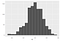Central Limit Theorem (CLT)

Central Limit Theorem states that if we take multiple random samples out of a population the distribution of their sample means will always be Normal. In descriptive statistics only a single sample is taken from the population and calculations are made based on it. However the results are suboptimal and are far from the population statistics. Therefore, in CLT

1. We take multiple samples of size(n) > 30 from the population. (sample size must always be => 30 observations)
2. Calculate means of the sample.
3. The distribution of samples is called Sampling distribution and the distribution of sampling means is called sampling distribution of means.

The individual sample maybe having exponential or binomial distribution but sampling distribution of means will always output normal distribution. Therefore we can say that the output distribution after application of the Central Limit Theorem is always Normal.Normal distribution of means of 1000 samples each of size 41 using Monte Carlo Simulation in R

The mean of the sampling distribution of means will always give a mean which is an accurate representation of the population mean.